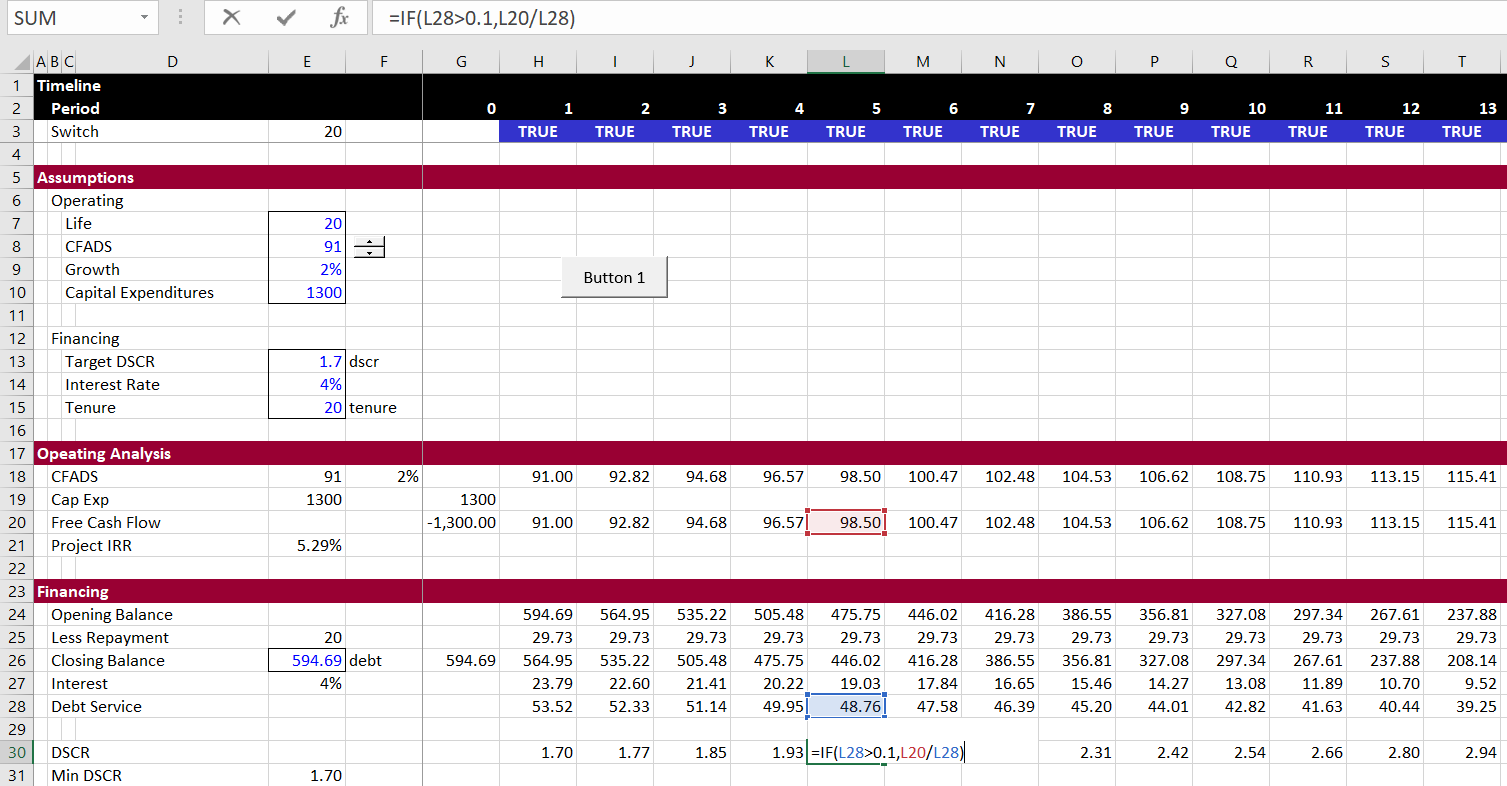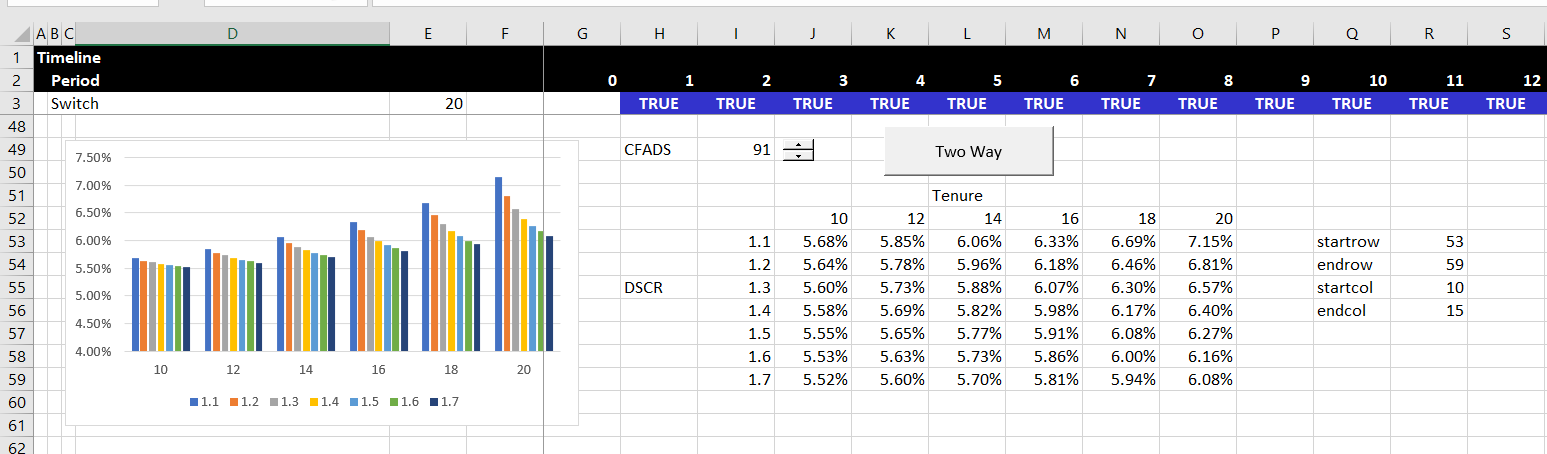# Data Tables & Goal Seek

This page demonstrates how to create a one-way and a two way data table using VBA where the result of each scenario depends on a goal seek.  The data table does not realise that it is supposed to run a goal seek each time a new result is put into the table. Using VBA code allows you to evaluate different scenarios and present scenario tables in different formats where the final results require a goal seek function. This webpage includes discussion of the VBA code with a FOR/NEXT function and the CELLS function. A project finance model where the debt size is established with a goal seek function driven by the target DSCR is used as an example. The example is used to illustrate basics of creating a VBA program.

The file that is used in explaining how to create the data table with the goal seek is included in the spreadsheet that you can download by pressing the button below.  This file includes a very simple project finance model where the size of the debt depends on an input debt service coverage ratio (DSCR). As the repayment of debt is assumed to be very simple with a flat repayment, a simple mathematical formula cannot be used.  Instead the debt amount can be computed by achieving the target DSCR with a goal seek formula.  The file below includes three macros.  The first is a basic one line goal seek macro. The second is a simple one way data table without flexible row and column ranges.  The third is a two-way data table that can be moved with flexible row and columns.

The first screenshot shows a simple project finance model that is used to demonstrate the VBA code. A simple project finance model is created where the debt size is set from an input DSCR.  In the analysis, a time line, operating cash flow and a debt schedule is established. Note that the repayment on row 25 is flat and the period by period DSCR on line 30 increases by each period.  In the case shown in the screenshot the goal seek has been run and the target goal seek equals the modelled.The insert below is the macro for creating a simple goal seek.  You just turn your record button on and then record the macro.  Then you should use range names for the target (in the case below defined as dif) and the input cell that is changed.  Make sure the input has no formula — not even something like =5.  If you are trying to make a goal seek for IRR, it is much better to use the NPV at the target IRR as the target cell with the formula.  If you want to make a goal seek with dynamic goal seeks you can create a user defined function — see the excel section.

.

```Sub goalseek()
'
' goalseek Macro
'
Range("dif").goalseek Goal:=0, ChangingCell:=Range("debt")
End Sub

.```

The screenshot below shows illustrates the results of the goal seek.  The button is attached to the macro with the goal seeks shown below.  If you are starting I suggest that you first try a one way table as illustrated below.  You then only have to make one loop around the rows.

```.
.
Sub oneway()
For Row = 41 To 47
Range("dscr") = Cells(Row, 9)
goalseek
Cells(Row, 10) = Range("eqirr")
Next Row
End Sub```The next screenshot illustrates how you can create a two-way table that changes both the tenure of the debt and the DSCR. Note that when you change two variables there is an interaction.  To create this table you need two loops.  One loop around the rows and the second loop around the columns..

```Sub twoway()
For Row = Range("startrow") To Range("endrow")
Range("dscr") = Cells(Row, Range("startcol") - 1)
For Column = Range("startcol") To Range("endcol")
Range("tenure") = Cells(Range("startrow") - 1, Column)
goalseek
Cells(Row, Column) = Range("eqirr")
Next Column
Next Row
End Sub```

.

.